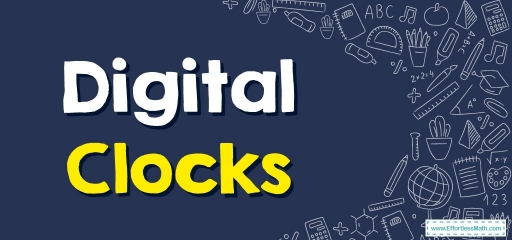# Digital ClocksDigital clocks are a kind that shows time digitally (i.e. via numbers or some other symbols), unlike analog clocks.

Reading the time on a digital form is extremely easy. With digital clocks, you’ll only see only $$2$$ numbers. The ones appearing on the left-hand side are the hours, and the ones appearing on the right-hand side are the minutes. A colon $$(:)$$ is used to separate hours from minutes.

## Related Topics

1- Read the $$1$$st numeral to ascertain the hour.
Digital clocks are made up of two numerals broken up by a colon. The $$1$$st numeral on digital clocks marks the hour.

• For instance, if the $$1$$st numeral is a “$$2$$,” it is in the $$2$$ o’clock hour.

2- Read the $$2$$nd numeral to ascertain the minutes. The $$2$$nd numeral on digital clocks, seen right after the colon, indicates the minutes into the hour.

• For instance, if it says “$$11$$,” it’s $$11$$ minutes into the hour.

3- Put both numerals together to find out what time it is. When you’ve read both numerals, you ought to know the time now. If a clock says “$$2:11$$,” that indicates the time is “two-eleven” or “eleven after two.

4- Decide if it is AM or PM. A few digital clocks indicate if it is AM or PM someplace on the clock. If not, just remember the time of day it is. Times get marked via AM if they are in-between midnight and noon. The times get marked as PM if they are in-between noon and midnight.

### Digital Clocks– Example 1:

What time is it? Write the time in words.

$$3:20$$

Solution:

As we mentioned, The $$1$$st numeral on digital clocks marks the hour, and the $$2$$nd numeral on digital clocks, seen right after the colon, indicates the minutes into the hour. So, the time is three twenty.

### Digital Clocks– Example 2:

What time is it? Write the time in words.

$$5: 17$$

Solution:

As we mentioned, The $$1$$st numeral on digital clocks marks the hour, and the $$2$$nd numeral on digital clocks, seen right after the colon, indicates the minutes into the hour. So, the time is five seventeen.

## Exercises for Digital Clocks

What time is it? Write the time in words.

1. $$\color{blue}{6:30}$$
2. $$\color{blue}{7:13}$$
3. $$\color{blue}{9:15}$$
4. $$\color{blue}{4:37}$$
1. It’s six thirty
2. It’s seven thirteen
3. It’s nine fifteen
4. It’s four thirty-seven

### What people say about "Digital Clocks - Effortless Math: We Help Students Learn to LOVE Mathematics"?

No one replied yet.

X
30% OFF

Limited time only!

Save Over 30%

SAVE $5 It was$16.99 now it is \$11.99